# Mathematics - Signals And Systems - Discrete-Time Fourier Series & Transform

in StemSocial2 months ago (edited)[Image1]

## Introduction

Hey it's a me again @drifter1!

Today we continue with my mathematics series about Signals and Systems in order to cover Discrete-Time Fourier Series & Transform.

So, without further ado, let's dive straight into it!

## Getting into Discrete-Time

In the previous three articles we covered how periodic and aperiodic continuous-time signals can be represented through the use of the Fourier Series and Fourier Transform correspondingly. In order to represent signals in Discrete-Time a similar principle is applied. In other words, such signals are again represented using linear combinations of complex exponentials. The complex exponentials are the eigenfunctions of LTI systems, which in turn simply allow for a complex amplitude change. Therefore, an LTI system is characterized by a spectrum of scale factors which are applied to each frequency.

## Discrete-Time Fourier Series

In Discrete-Time, a Fourier series is again constructed using harmonically related complex exponentials. The fundamental frequencies of those complex exponentials are multiples of the fundamental frequency of the periodic sequence that's to be represented.

In the article about complex exponentials we mentioned that complex exponentials are only unique when the frequency variables spans a range of 2π. Beyond this range, the complex exponential is repeated over and over. This is a very important difference that continuous- and discrete-time complex exponential signals have. As such, a periodic sequence with period N can be easily represented as a linear combination of complex exponentials of the form: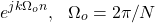as there are only N unique complex exponentials available.

Complex exponentials of this form are periodic in k with period N. Additionally, they are also periodic in n with period N.

Its easy to conclude that this simplifies the analysis part for Discrete-Time quite a bit, as only N Fourier series coefficients need to be determined. The whole problem is simplified into solving N equations of N unknowns.

### Synthesis equation

Using Fourier Series, a Discrete-Time period signal x[n] is represented as follows: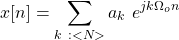where k : <N> is used for denoting a quantity of N coefficients.

As the number of coefficients used is increased, a signal can be approximated better and better, as more frequency scale factors are "discovered". The GIF that follows demonstrates this for a square wave: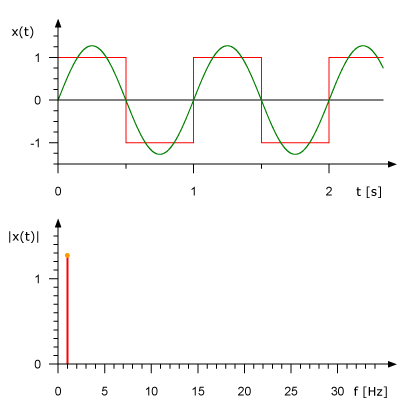[Image 2]

### Analysis equation

The analysis part is about calculating the correct ak coefficients. In total there are N equations of the form: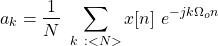## Discrete-Time Fourier Transform

In order to represent an aperiodic signal, a similar approach to Continuous-Time is used. So, we construct a periodic signal of x[n], which has a Fourier Series. As the period of this periodic signal increases its Fourier Series turns into the Fourier Transform of the original signal x[n].

Mathematically: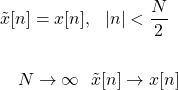Similar to Continuous-Time, the coefficients will be taken as samples of an envelope. The envelope is determined by the behavior of a sequence over one period, but not by the specific value of the period. Increasing the period of the sequence increases, the nonzero values inside of one period remain the same. So, its easy to conclude that the Fourier series coefficients are samples of the same envelope function, but with increasingly finer spacing along the frequency axis. This envelope function allows for the representation of an aperiodic signal in one period, giving us the Fourier Transform of the aperiodic signal.

### Fourier series of periodic x[n]

The periodic x[n] can be represented using a Fourier Series, as follows: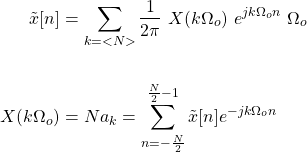### Synthesis equation

As N → ∞ the first sum turns into an integral, and converges to x[n], giving us the Fourier Transform synthesis equation: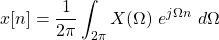### Analysis equation

As N → ∞ in the coefficient calculation, the equation gives us the Inverse Fourier Transform or analysis equation: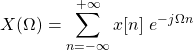The Fourier Transform turns any aperiodic discrete-time signal x[n] into its envelope samples X(Ω), and vise versa: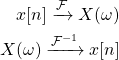### Fourier Transform of periodic signal

The Fourier Transform can also be defined for periodic signals.

Similar to Continuous-Time, the Fourier Series coefficients are 1 / N times the samples of the Fourier Transform (in CT it was 1 / To) and defined using an impulse train: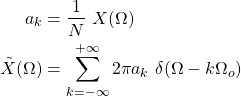## RESOURCES:

### Images

Block diagrams and other visualizations were made using draw.io

## Final words | Next up

And this is actually it for today's post!

Next time we will cover the Properties of the Discrete-Time Fourier Transform!

See Ya!Keep on drifting!